• 图像傅里叶变换的频谱特征 6，平移和旋转 图像的平移并不会影响图像的频谱，同时，图像的相位会随着图像的旋转而旋转。 Part I 平移和旋转对频谱的影响 下面我用矩形的频谱图来说明图像中矩形的平移并...
                               图像傅里叶变换的频谱特征 三

6，平移和旋转
图像的平移并不会影响图像的频谱，同时，图像的相位会随着图像的旋转而旋转。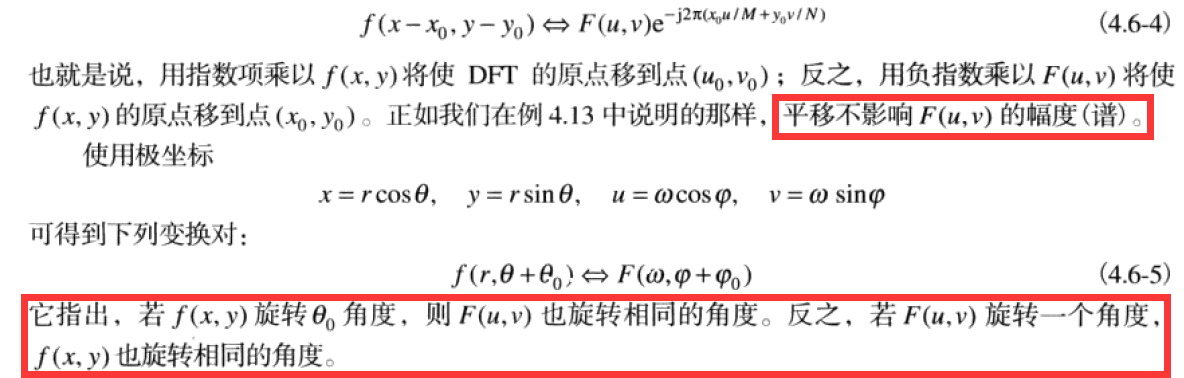Part I 平移和旋转对频谱的影响
下面我用矩形的频谱图来说明图像中矩形的平移并不会对频谱有丝毫的影响。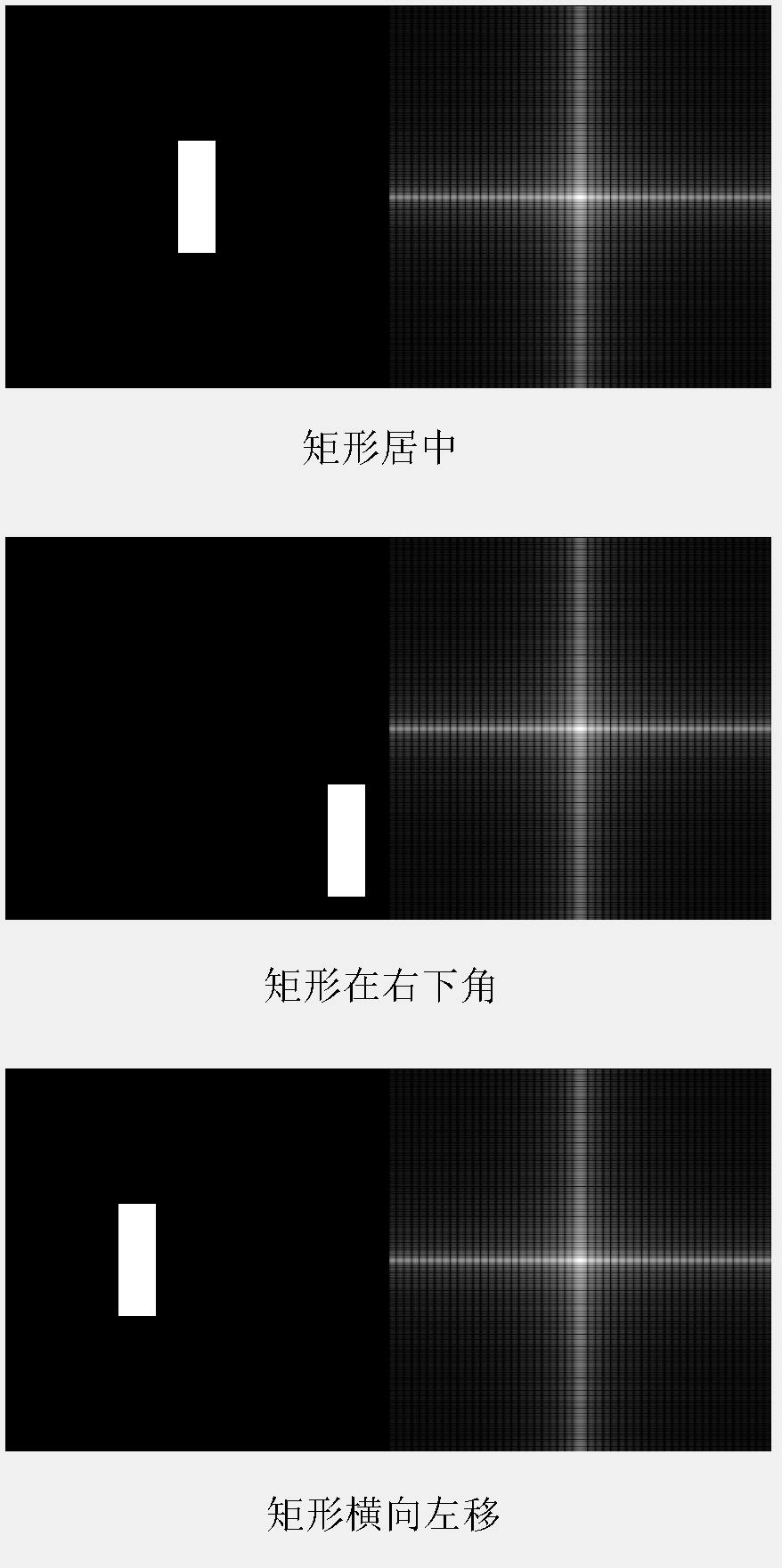Matlab代码：

clear all
close all
%% Author: J27
% Jesus love you!

Isize = 512;
Rwidth = 50;
Rlength = 3*Rwidth;

Irect = zeros(Isize);
Irect(floor((Isize - Rlength)/2) + 1:floor((Isize - Rlength)/2) + Rlength,...
(floor(Isize - Rwidth)/2) + 1:floor((Isize - Rwidth)/2) + Rwidth) = 1;

subplot(3,1,1)
imshowpair(Irect,log(abs(fftshift(fft2(Irect)))+1),'montage')

Irect = zeros(Isize);
Irect(floor((Isize - Rlength)/2) + 150 + 1:floor((Isize - Rlength)/2) + 150 + Rlength,...
(floor(Isize - Rwidth)/2) + 1 + 200:floor((Isize - Rwidth)/2) + Rwidth + 200) = 1;

subplot(3,1,2)
imshowpair(Irect,log(abs(fftshift(fft2(Irect)))+1),'montage')

Irect = zeros(Isize);
Irect(floor((Isize - Rlength)/2) + 1:floor((Isize - Rlength)/2) + Rlength,...
(floor(Isize - Rwidth)/2) + 1 - 80:floor((Isize - Rwidth)/2) + Rwidth - 80) = 1;

subplot(3,1,3)
imshowpair(Irect,log(abs(fftshift(fft2(Irect)))+1),'montage')

再比如下面这个例子：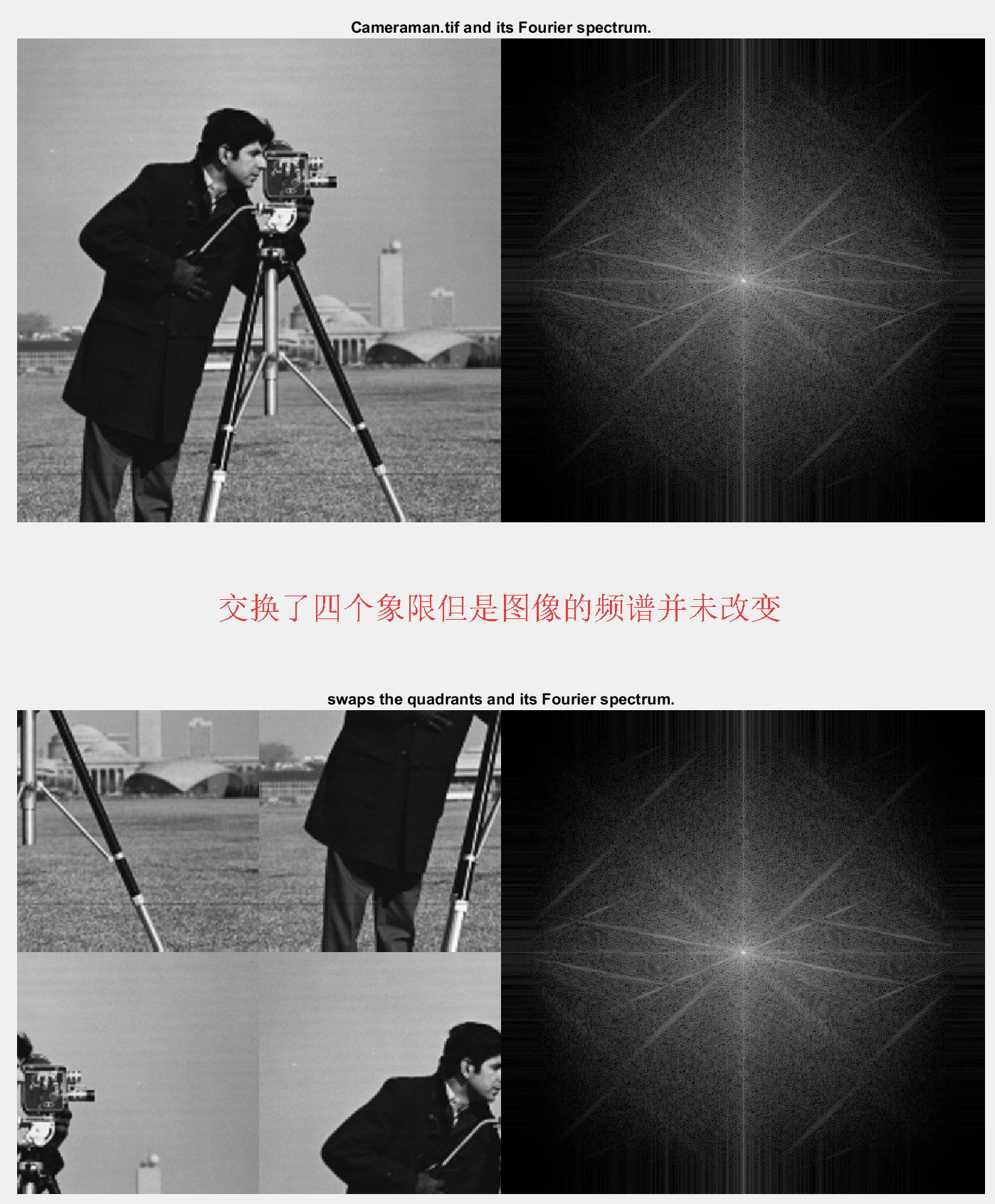再来看看频谱随着矩形的旋转而旋转相同的角度。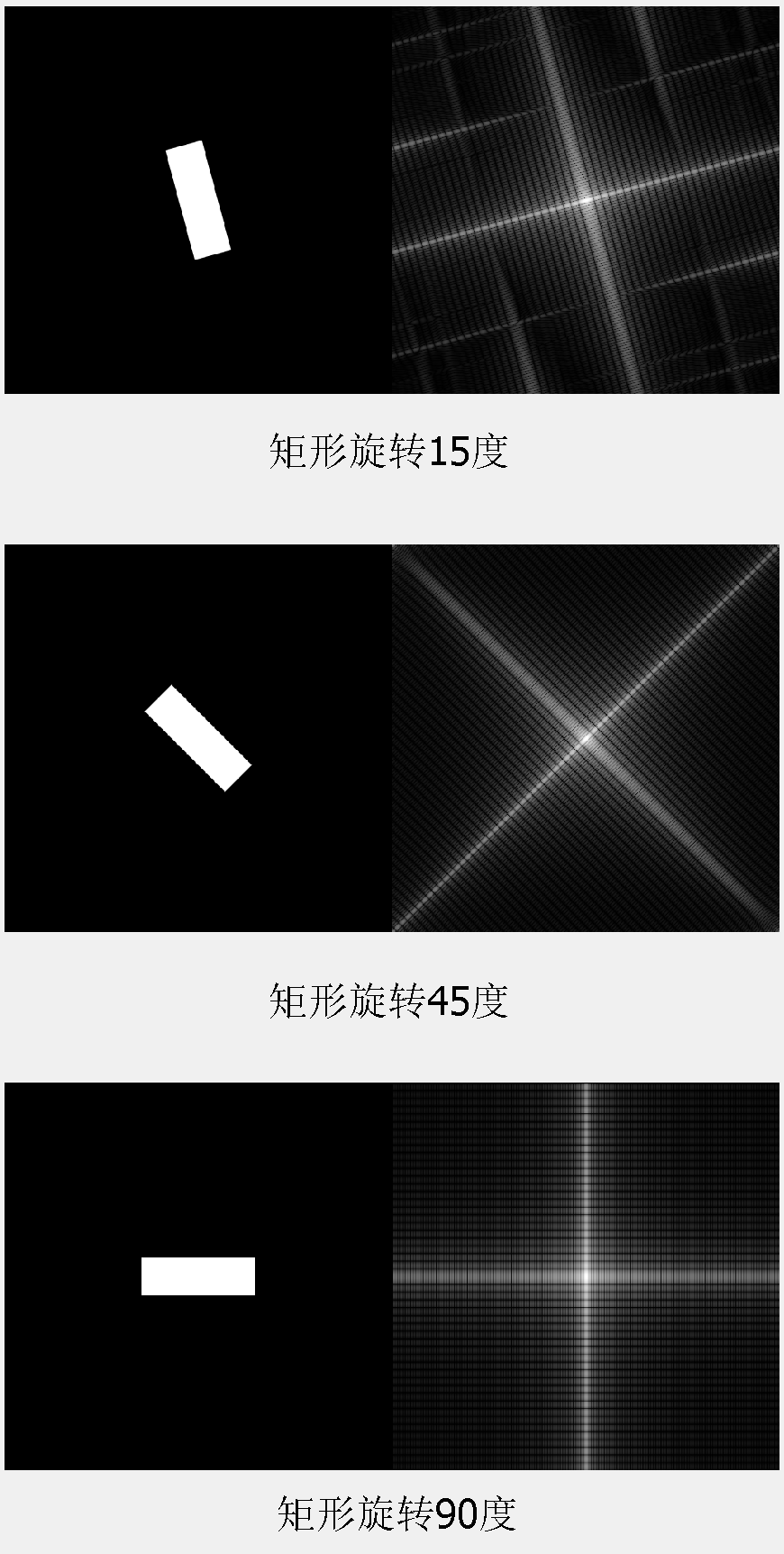Matlab代码：
Isize = 512;
Rwidth = 50;
Rlength = 3*Rwidth;
Irect = zeros(Isize);

Irect(floor((Isize - Rlength)/2) + 1:floor((Isize - Rlength)/2) + Rlength,...
(floor(Isize - Rwidth)/2) + 1:floor((Isize - Rwidth)/2) + Rwidth) = 1;

Irot = imrotate(Irect, 15, 'crop', 'bilinear');
subplot(3,1,1)
imshowpair(Irot,log(abs(fftshift(fft2(Irot)))+1),'montage')

Irect = zeros(Isize);
Irect(floor((Isize - Rlength)/2) + 1:floor((Isize - Rlength)/2) + Rlength,...
(floor(Isize - Rwidth)/2) + 1:floor((Isize - Rwidth)/2) + Rwidth) = 1;

Irot = imrotate(Irect, 45, 'crop', 'bilinear');
subplot(3,1,2)
imshowpair(Irot,log(abs(fftshift(fft2(Irot)))+1),'montage')

Irect = zeros(Isize);
Irect(floor((Isize - Rlength)/2) + 1:floor((Isize - Rlength)/2) + Rlength,...
(floor(Isize - Rwidth)/2) + 1:floor((Isize - Rwidth)/2) + Rwidth) = 1;

Irot = imrotate(Irect, 90, 'crop', 'bilinear');
subplot(3,1,3)
imshowpair(Irot,log(abs(fftshift(fft2(Irot)))+1),'montage')

Part II 平移和旋转对相位的影响
先用一个简单的例子来说明图像相位的作用（所用图像为cameraman），在图像的频域分析和滤波中，相位是常常被忽略的。虽然相位分量的贡献很不直观，但是它恰恰很重要。相位是频谱中各正弦分量关于原点的位移的度量。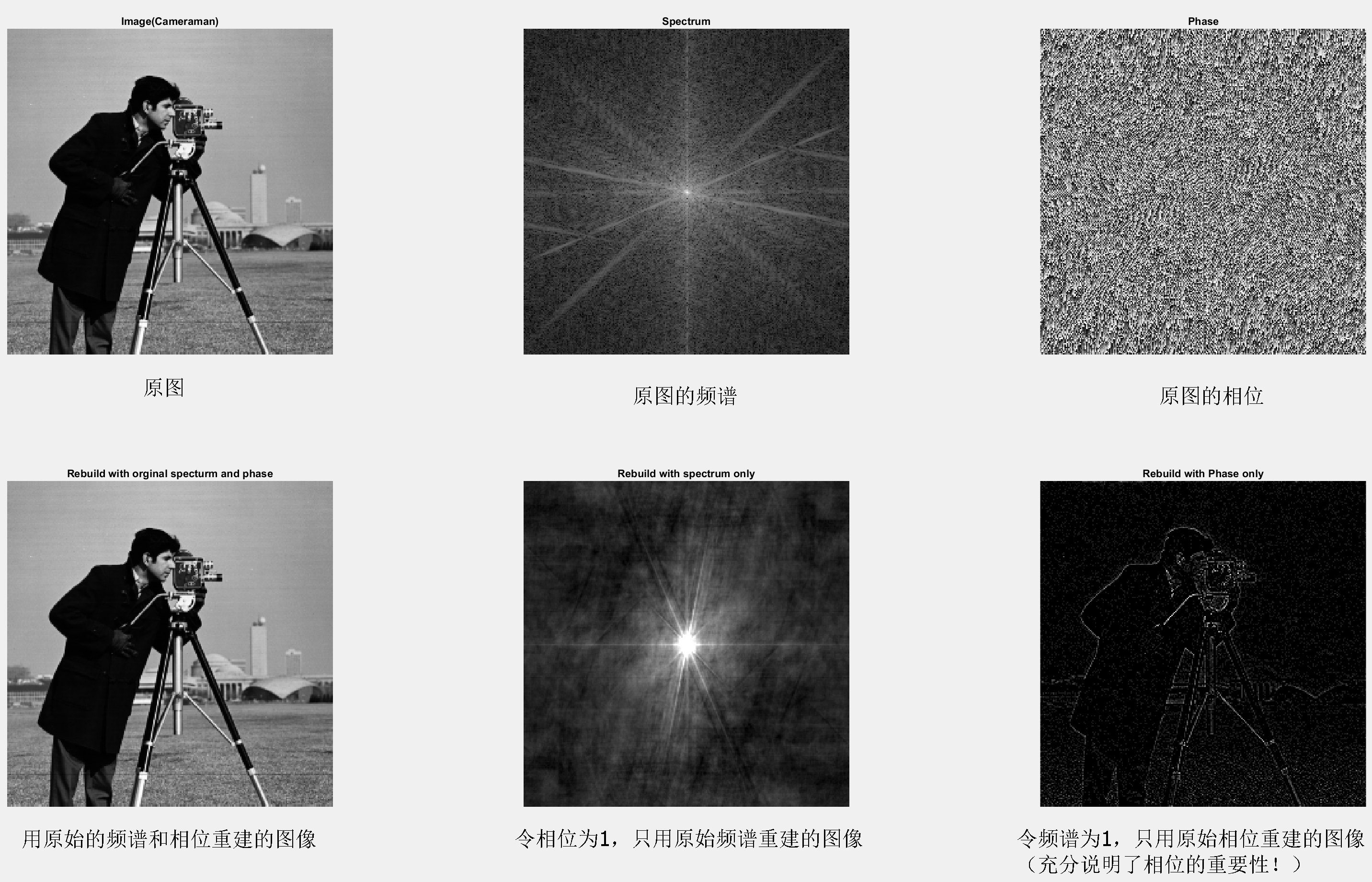上面的小实验充分说明了，看似无用的，且常常被忽略的相位，在DFT的频域中起到了多么重要的作用（注意区分实部和虚部（直角坐标系）VS 频谱和相位（极坐标系）！）。
Matlab代码：
close all;
clear all;
DFT = fft2(I);
absF = abs(DFT);
Phi = atan2(imag(DFT),real(DFT));
subplot(2,3,1);
imshow(I,[]);
title('Image(Cameraman)');
subplot(2,3,2);
imshow(log(fftshift(absF) + 1),[]);
title('Spectrum');
subplot(2,3,3);
imshow(fftshift(Phi),[]);
title('Phase');

F = absF.*exp(1j*Phi);
Rebuild = im2uint8(real(ifft2(F)));
subplot(2,3,4);
imshow(Rebuild,[]);
title('Rebuild with orginal specturm and phase');
% rebuild with spectrum only
F = absF.*exp(1j*1);
Rebuild = im2uint8(real(fftshift(ifft2(F))));
subplot(2,3,5);
imshow(Rebuild,[]);
title('Rebuild with spectrum only');
% rebuild with Phase only
g = 1.*exp(1j*Phi);
g = im2uint8(real(ifft2(g)));
subplot(2,3,6);
imshow(g,[]);
title('Rebuild with Phase only');
接下来我们再来看看图像在空间域中的移位和旋转对相位有什么影响。下图中，左边一列是图像，中间一列是频谱，右边一列是相位图。你必须意识到，通过肉眼，你很难从相位图中得到什么有用的信息。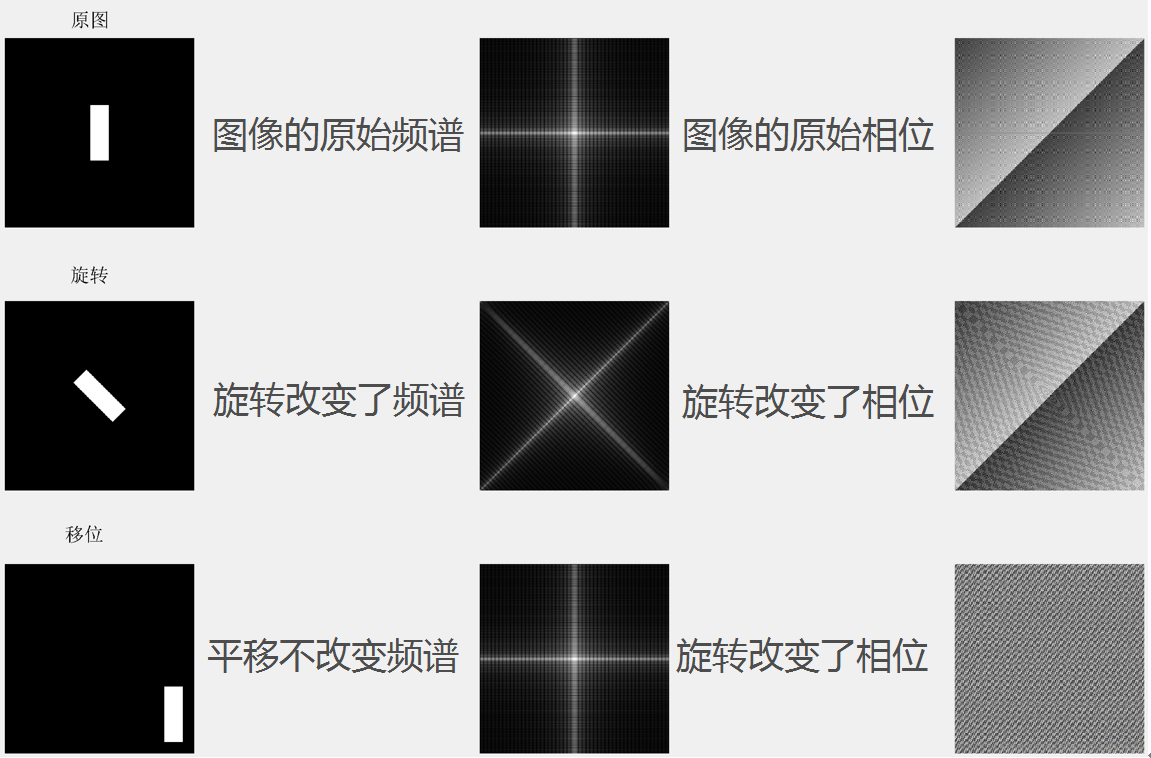(上图中最后一行打错了，不是“旋转改变了相位”而是“平移改变了相位”)
Matlab代码：
clear all
close all
%% Author: J27
% Jesus love you!

% shifting and rotation
Isize = 512;
Rwidth = 50;
Rlength = 3*Rwidth;

Irect = zeros(Isize);
Irect(floor((Isize - Rlength)/2) + 1:floor((Isize - Rlength)/2) + Rlength,...
(floor(Isize - Rwidth)/2) + 1:floor((Isize - Rwidth)/2) + Rwidth) = 1;
Idft = fft2(Irect);
subplot(3,3,1);
imshow(Irect);
subplot(3,3,2);
imshow(log(abs(fftshift(Idft))+1),[]);
subplot(3,3,3);
imshow((atan2(imag(Idft),real(Idft))),[]);

Irot = imrotate(Irect, 45, 'crop', 'bilinear');
Idft = fft2(Irot);
subplot(3,3,4);
imshow(Irot);
subplot(3,3,5);
imshow(log(abs(fftshift(Idft))+1),[]);
subplot(3,3,6);
imshow(atan2(imag(Idft),real(Idft)),[]);

Irect = zeros(Isize);
Irect(floor((Isize - Rlength)/2) + 150 + 1:floor((Isize - Rlength)/2) + 150 + Rlength,...
(floor(Isize - Rwidth)/2) + 1 + 200:floor((Isize - Rwidth)/2) + Rwidth + 200) = 1;
Idft = fft2(Irect);
subplot(3,3,7);
imshow(Irect);
subplot(3,3,8);
imshow(log(abs(fftshift(Idft))+1),[]);
subplot(3,3,9);
imshow(atan2(imag(Idft),real(Idft)),[]);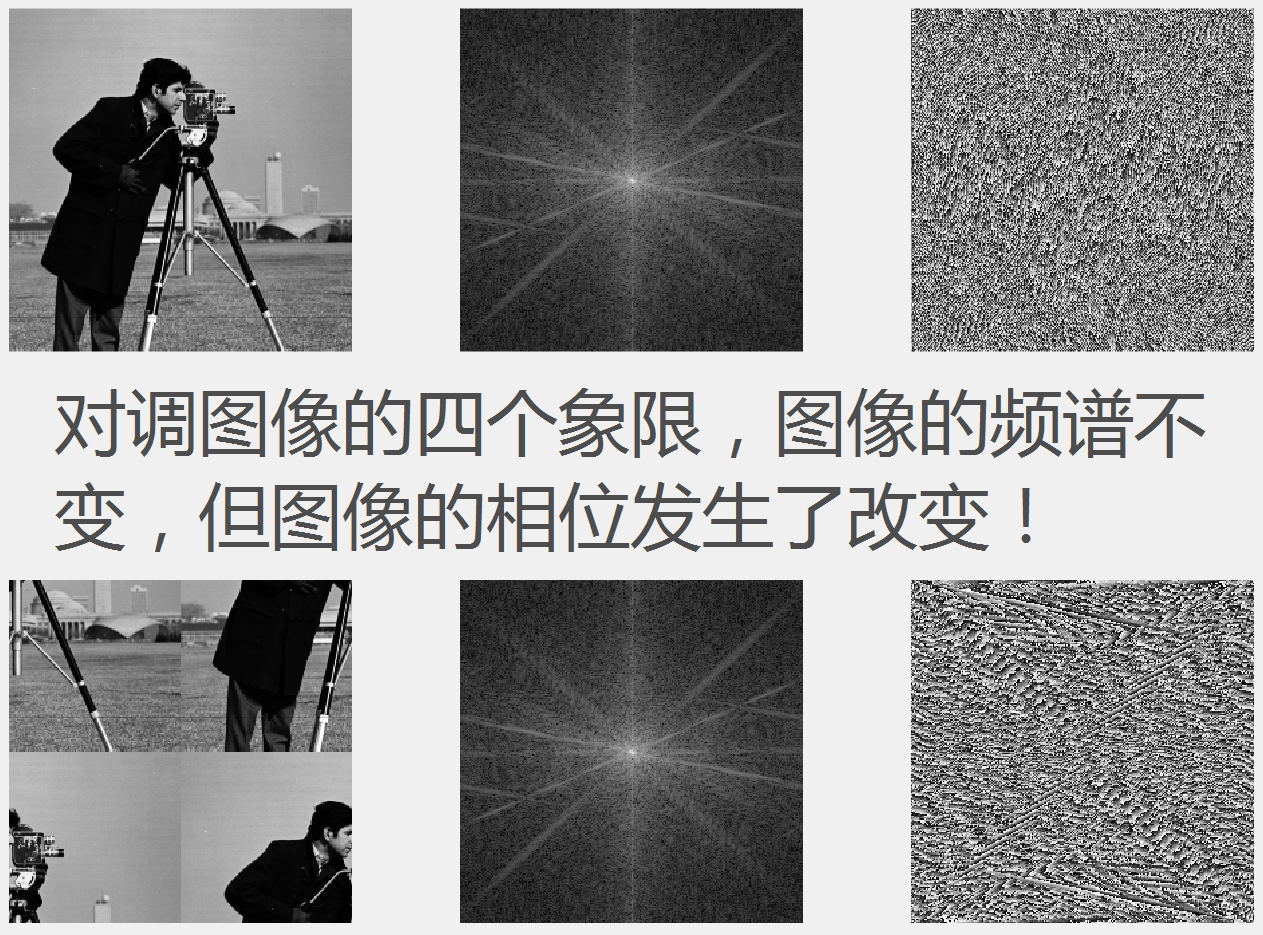Matlab代码：
I = im2double(imread('cameraman.tif'));
Idft = fft2(I);
figure;
subplot(2,3,1);
imshow(I,[]);
subplot(2,3,2);
imshow(log(abs(fftshift(Idft))+1),[]);
subplot(2,3,3);
imshow(atan2(imag(Idft),real(Idft)),[]);

Iswap = fftshift(I);
Idft = fft2(Iswap);
subplot(2,3,4);
imshow(Iswap,[]);
subplot(2,3,5);
imshow(log(abs(fftshift(Idft))+1),[]);
subplot(2,3,6);
imshow(atan2(imag(Idft),real(Idft)),[]);
（全文完）
谢谢收看！

《圣经》约翰福音15章5节 ------ 我是葡萄树，你们是枝子；常在我里面的，我也常在他里面，这人就多结果子；因为离了我，你们就不能作什么。*配图与本文无关*
展开全文二维傅里叶变换
• 通过对平移断裂的展布、形态、伴随构造、牵引构造、位移方向和幅度、平移时间、断层尾端及动力学演化等特征研究,认为该断裂主要活动于晚叠世,在晚侏罗世-早白垩世最为活跃,伴随岩浆侵入活动、火山喷发及陆相沉积。...
• 根据可视化需求，将建筑分为三个层次，在此基础上，以最短距离为指标实现了平行结构建筑的面平移化简算法。实验证明，该算法具有较高的效率和较好的通用-胜，对于平行结构建筑几何特征的化简具有良好的效果。
• 精品文档 第章 图形的平移与旋转 知识点一平移平移作图 1平移的概念及性质 (1) 平移的概念在平面内将一图形沿某个方向移动一定的距离这样的图形运动 称为平移平移不改变图形的形状和大小 2平移特点 图形上的...
• 平移的概念在平面内将一图形沿某个方向移动一定的距离这样的图形运动 称为平移平移不改变图形的形状和大小 2平移特点 图形上的每一点都沿同一方向移动相同的距离 平移不改变图形的形状大小方向只改变图形的...
• 镜像变换1.3 剪切变换1.4 旋转变换1.5 平移变换1.5.1 原本平移变换1.5.2 平移变换齐次坐标表示1.6 逆变换1.7 组合变换1.8 非原点的旋转变换1.9 二维主要变换矩阵总结1.10 二维矩阵变换合并2 维变换2.1 平移...


图形学中的基本变换
1. 二维变换1.1 缩放变换1.2.镜像变换1.3 剪切变换1.4 旋转变换1.5 平移变换1.5.1 什么是线性变换1.5.2 平移变换(仿射变换)1.5.3 平移变换齐次坐标表示(线性)
1.6 逆变换1.7 组合变换1.8 非原点的旋转变换1.9 刚体变换1.10 二维主要变换总结
2 三维变换2.1 三维平移变换2.2 三维缩放矩阵2.3 三维旋转矩阵2.3.1 绕z轴旋转矩阵2.3.2 绕x轴旋转矩阵2.3.3 绕y轴旋转矩阵2.3.4 绕任意轴旋转矩阵

1. 二维变换
1.1 缩放变换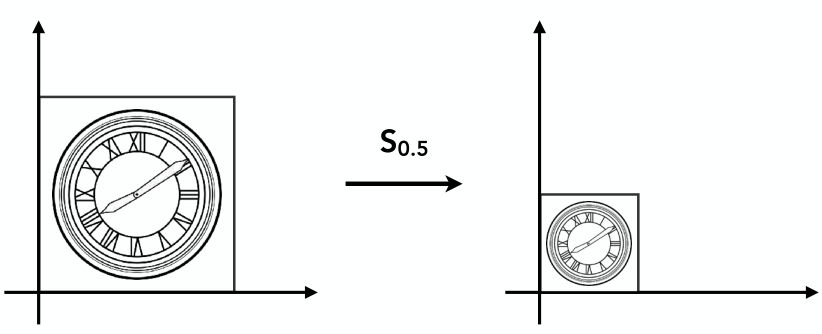如上图，如果想把一个图形缩小为原来的0.5倍，那么就需要x坐标变为0.5倍，y坐标也变为0.5倍，可以用以下表达式表示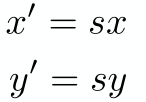前面两节已经学了一些矩阵方面的知识，那么这两个表达式可以用矩阵的形式表示如下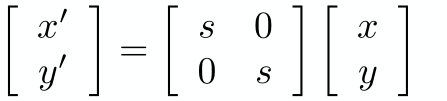上面的矩阵表达式针对x轴和y轴进行相同比例的缩放，实际中两个方向上的缩放可能不尽相同，例如x轴缩放为0.5倍与轴不缩放，这时只需要把矩阵表达式稍作修改即可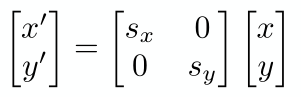Sx表示在x轴方向上缩放的倍数，Sy表示在y轴方向上缩放的倍数
1.2.镜像变换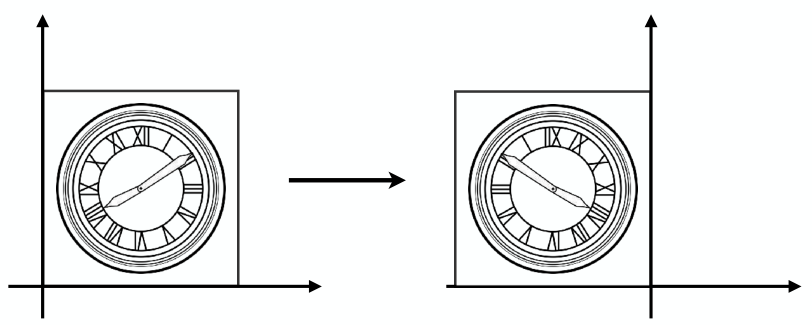如上图需要将物体以y轴进行镜像，那么可以用以下表达式表达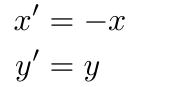也可以用矩阵形式的表达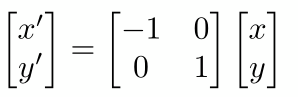一些其他镜像矩阵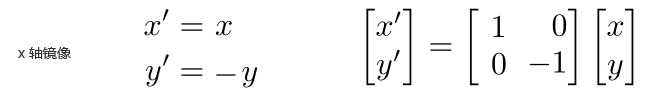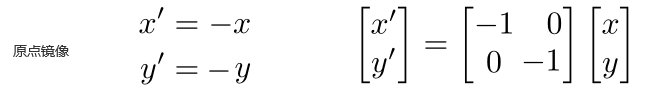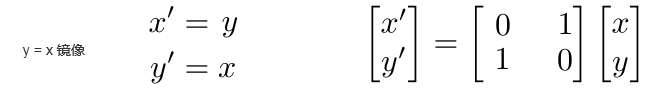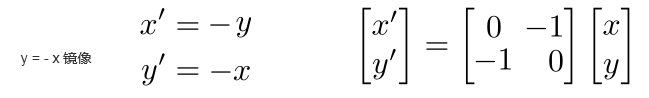1.3 剪切变换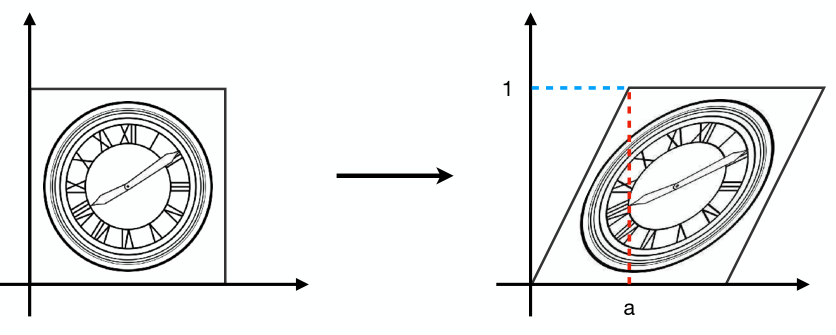如上图这个变换好像是拽着图形的右上角沿着x轴向右拉了一段距离，称为剪切变换。 剪切变换有以下特点
变换后物体的y坐标保持不变x坐标在最高的点平移了a，最低点没有移动其它的点移动距离a * Y (Y表示物体上点的y坐标值)
用矩阵形式的表达为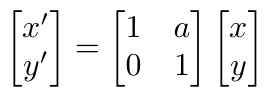1.4 旋转变换
说旋转，默认指的是 绕原点(0,0)逆时针旋转，下图是物体绕原点逆时针旋转θ角的示意图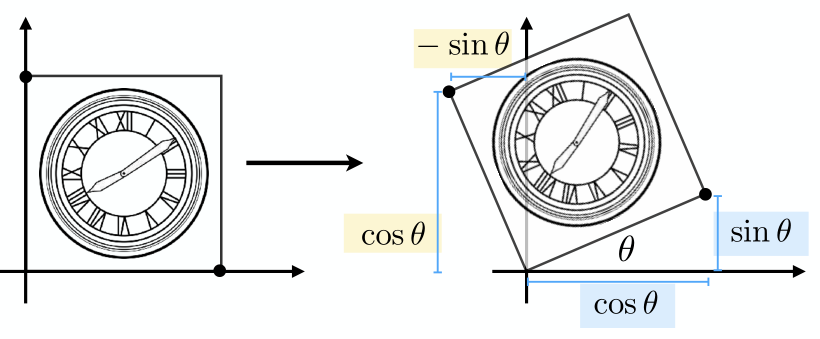以上变换同样可以写成矩阵的形式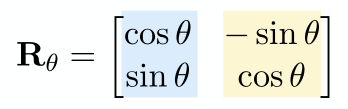1.5 平移变换
1.5.1 什么是线性变换
什么是线性变换，前面提到的变换都可以使用以下表达式表示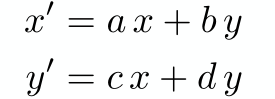都可以使用矩阵形式表示为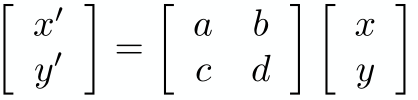继而表示为：输出坐标 = 变换矩阵 × 输入坐标 的形式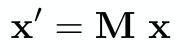满足以上条件的变换称为 线性变换
1.5.2 平移变换(仿射变换)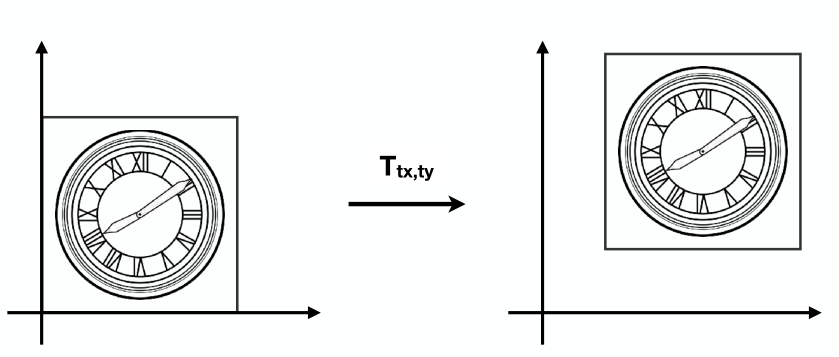如上图需要把一个图形沿x轴平移tx，沿y轴平移ty，可以用以下表达式表示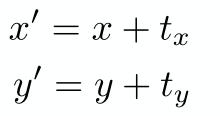你会发现，它 无法用前面熟悉的线性变换矩阵的形式表示，也就是说平移变换是非线性变换
只能用以下矩阵形式表示，上面把这种变换称为非线性变换，其实它有专门的名字叫仿射变换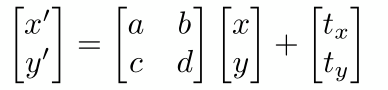人们总是希望能有一个统一的方法来表示同类事物，希望平移变换也能够像其他变换一样用同样的形式表示，使用起来更加便捷统一。
前辈大牛们经过各种尝试，想出了一个办法，就是引入齐次坐标
1.5.3 平移变换齐次坐标表示(线性)
什么是齐次坐标
齐次坐标与笛卡尔坐标系相比，会比笛卡尔坐标系多出一维，例如原来的坐标系是二维，转为齐次坐标系后就会变成三维，如果在卡尔坐标系上有点(x,y);当转换为齐次坐标后这个点变为(wx,wy,w);反过来同样适用，如果在其次坐标系中有一个点(x,y,w)，转换到笛卡尔坐标系下，这个点应该表示为(x/w,y/w),一个点在齐次坐标下有无数的表示法，例如笛卡尔坐标系下有点(1,2)，在其次坐标系下它可以表示为(1,2,1)、(2,4,2)、(3,6,3) …
点和向量转为齐次坐标表示
点(x,y)转为齐次坐标表示为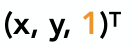向量(x,y)转为齐次坐标表示为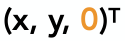这里解释一下在转为齐次坐标的过程中，点在末尾添加1，而向量添加0。 向量具有平移不变形，任何一个向量平移后表示原来的向量，在向量转为齐次坐标形式的时候，在末尾添加0，就是为了保护这个向量在平移过程中不发生变化。
平移变换齐次坐标表示
平移变换原本是仿射变换，通过引入齐次坐标后，可以使用线性变换的形式表示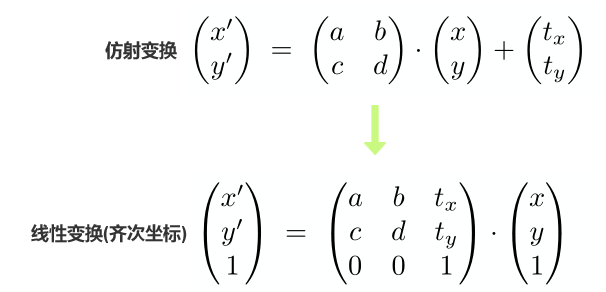这样我们的目的就达到了，把平移变换也表示为一个矩阵乘以一个坐标的线性变换的形式
1.6 逆变换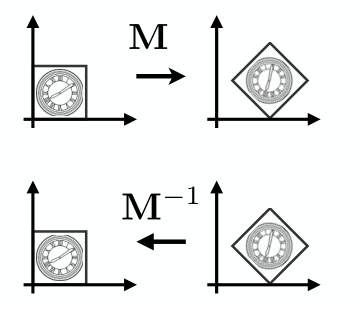一个物体做一个变换，变换完以后要恢复到原来的位置，变换回原来的位置的过程称为逆变换，逆变换在数学上的实现是乘以变换矩阵的 逆矩阵
1.7 组合变换
组合变换就是对一个物体进行多个变换，例如同时进行平移和旋转变换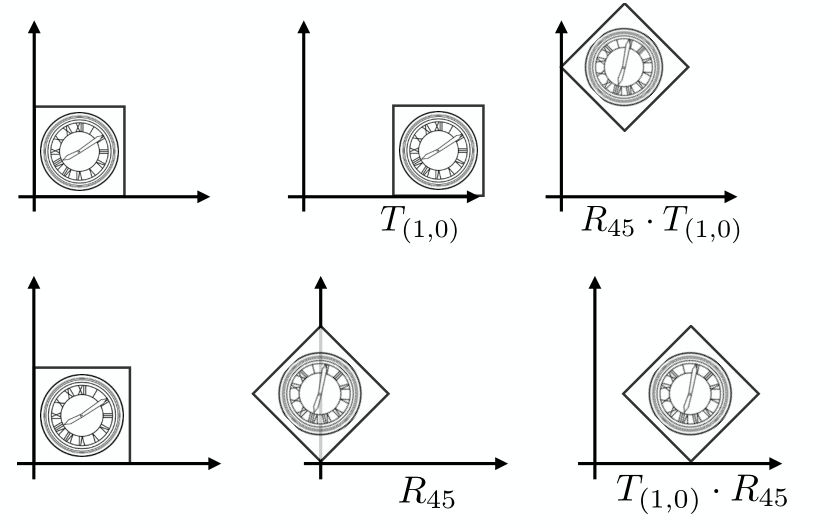上图中有两套组合变换
先平移(1,0)，再旋转45度先旋转45度，再平移(1,0)
你会发现虽然都作了相同的变换，但是变换的顺序不同最终的结果也不同，是因为矩阵A乘矩阵B与矩阵B乘矩阵A的结果不同(上一节学习的矩阵相乘性质)
组合变换矩阵相乘应用的顺序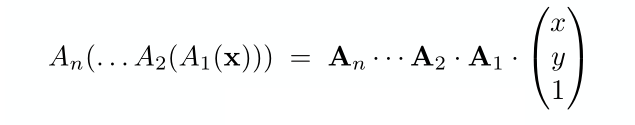上图中A1，A2一直到An表示变换矩阵，一个点进行组合变换时，应用在该点的矩阵是从右到左。即矩阵An乘An-1一直乘到A1，实际应用到点的顺序是A1，A2一直到An
矩阵乘法结合律使用 之前的学习中我们知道矩阵相乘交换律不适用，但是适用结合律，既然适用有什么妙用，接下来看看 一个点做多个变换即多个矩阵相乘再乘以这个点，根据矩阵乘法结合律，可以先把这些矩阵相乘，乘完在与这个点相乘，只要保证矩阵相乘的顺序不变即可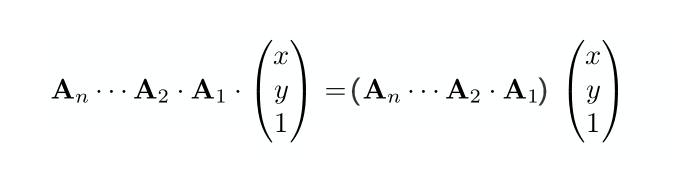1.8 非原点的旋转变换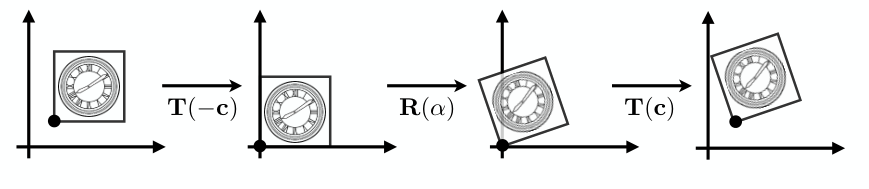我们学会了物体绕原点旋转，对于一个不是绕原点旋转的变换需要换个思维实现，实现过程分三步
将物体旋转要绕的点移动到原点，移到原点后做旋转变换旋转变换完成后平移回原来的位置
这个过程用一个表达式表示矩阵变换作用在物体上的顺序是从右到左，所以上面表达式表示，先平移T(-c)到原点,然后旋转R(α)，最后平移到原来位置T（c），一定要注意先后顺序
1.9 刚体变换
只有平移和旋转组成的变换称为刚体变换，例如一个物体先旋转45度在x轴方向上平移一个单位，这样的变换称为刚体变换，刚体变换的本质是一个物体的位置和角度发生了变换，物体本身的形状并不发生任何变化 假如有一个刚体变换先逆时针旋转45度，在沿x轴平移1个单位，那么这个变换可以用下面的矩阵表示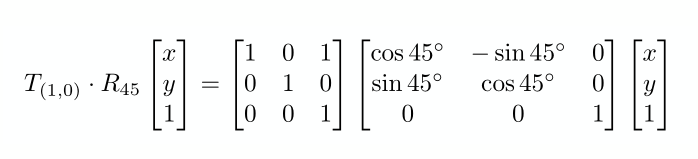上面的变换是先进行线性变换-旋转，在进行仿射变换-平移，这时可以把两个变换的矩阵合并为一个矩阵，之所以可以合并因为在同一个矩阵同时表示两种变换时，会先进行线性变换再进行仿射变换，这与我们提到的变换顺序是一致的，两种变换合并为一个矩阵用来表示刚体变换，这个矩阵称为刚体变换矩阵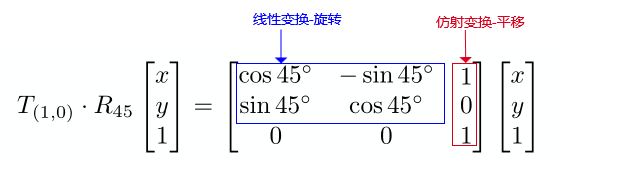刚体变换的逆变换 二维刚体变换的逆变换矩阵，只需要把原变换矩阵左上角2×2矩阵(上图蓝色框部分)转置，右侧最后一列(上图红色框部分)的平移分量符号取反。就可以得到刚体变换的逆变换矩阵
1.10 二维主要变换总结
图形变换中的三大变换为缩放、旋转、平移。下面把这三类变换的变换矩阵罗列一下，方便日后使用
缩放矩阵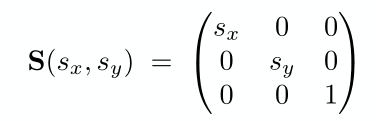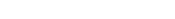旋转矩阵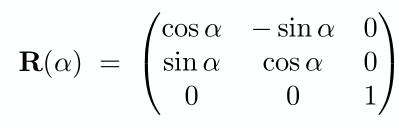上述旋转矩阵是绕原点逆时针旋转的变换矩阵，当需要得到顺时针旋转的变换矩阵时，可以通过顺时针旋转的变换矩阵进行逆变换得到逆时针旋转的变换矩阵，即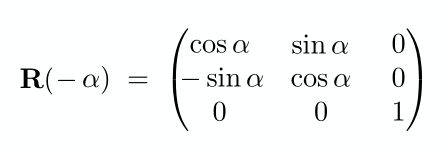你会发现这个矩阵不就是逆时针旋转变换矩阵的转置矩阵，没错就是这样的，旋转变换矩阵的逆矩阵与转置矩阵就是同一个矩阵。像这样一个矩阵的转置等于这个矩阵的逆，我们称这个矩阵是 正交矩阵平移矩阵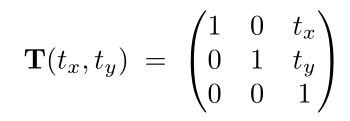2 三维变换
2.1 三维平移变换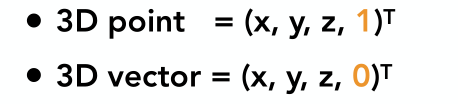三维坐标系转为齐次坐标，与二维坐标系转为齐次坐标类似，一个点在末尾添加1，一个向量添加0。
二维平移矩阵是仿射变换，可以转换到齐次坐标下使用线性变换的形式表示，三维平移矩阵也是仿射变换，同样的道理也可以转换到齐次坐标下用线性变换矩阵的形式表示，下图就是齐次坐标下三维平移变换矩阵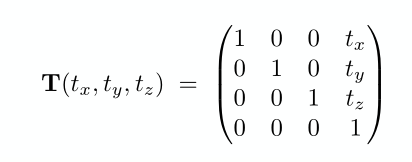2.2 三维缩放矩阵
我们知道了而是缩放矩阵了，那么推理三维缩放矩阵非常简单，就是在矩阵中增加一维z分量即可，三维缩放矩阵就可以表示如下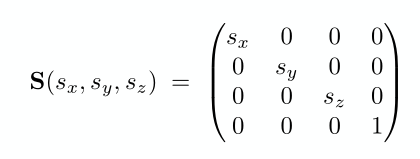2.3 三维旋转矩阵
2.3.1 绕z轴旋转矩阵
绕z轴旋转矩阵的推导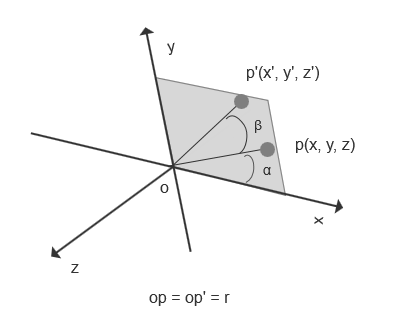如上图，若有一点p在x轴和y轴所在平面上，p到到原点o的距离为r，即op=op’=r；p点到原点o的连线与x轴的夹角是α，p点绕z轴旋转β角，p点旋转到p’点的位置，则可以得到以下关系
x = cos(α)*r
y = sin(α)*r
x' = cos(α+β)*r
y' = sin(α+β)*r

三角函数两角求和公式
cos(α+β) = cos(α)*cos(β) - sin(α)*sin(β)
sin(α+β) = sin(α)*cos(β) + cos(α)*sin(β)

使用上面的关系和三角函数求和公式得到
x' = cos(α+β)*r
= (cos(α)*cos(β) - sin(α)*sin(β))*r
= (cos(α)*r)*cos(β) - (sin(α)*r)*sin(β) //将x1 = cos(α)*r 和 y1 = sin(α)*r 带入
= x*cos(β) - y*sin(β)

y' = sin(α+β)*r
= (sin(α)*cos(β) + cos(α)*sin(β))*r
= (sin(α)*r)*cos(β) + (cos(α)*r)*sin(β) //将x1 = cos(α)*r 和 y1 = sin(α)*r 带入
= y*cos(β) + x*sin(β)
= x*sin(β) + y*cos(β)

z' = z

上面得到的结果是绕z轴旋转β角的结果，把β替换为α则有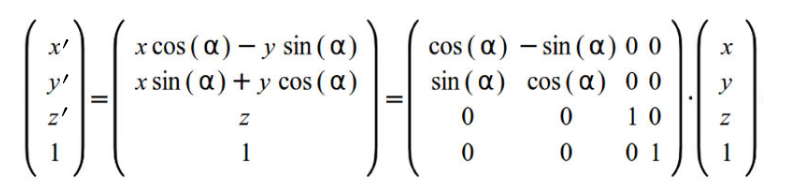最终得到绕z轴旋转α角的旋转矩阵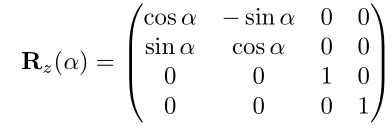2.3.2 绕x轴旋转矩阵
推导方法参照绕z轴旋转矩阵推导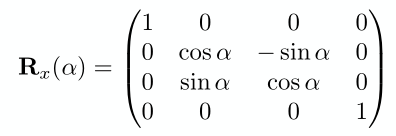2.3.3 绕y轴旋转矩阵
推导方法参照绕z轴旋转矩阵推导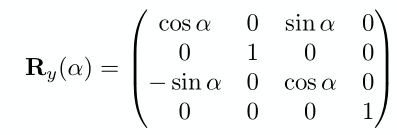2.3.4 绕任意轴旋转矩阵
绕任意轴旋转可分解为绕x轴旋转α角，绕y轴旋转β角，绕z轴旋转γ角，用以下形式表示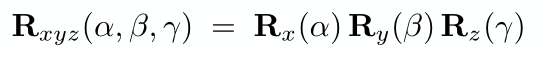将绕绕三轴旋转转化为绕一个单位向量n旋转α角，向量n默认穿过原点，用下面的矩阵表示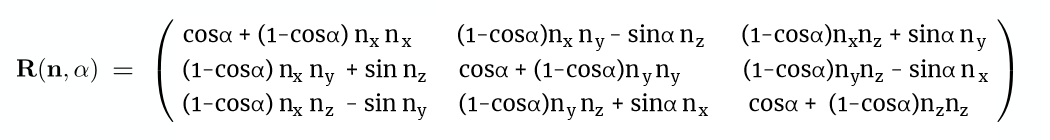上面这个公式只适用于绕穿过原点的向量旋转变换，如果遇到绕任意轴时怎么办？ 在二维空间中旋转若不是绕原点旋转，需要先平移到原点，再作旋转，最后要平移回原来的位置，三维空间中绕未穿原点的向量旋转，可以先将旋转轴平移至穿过原点，进行旋转，旋转完成后再进行平移的逆变换，将物体移回原来位置
​
​
展开全文计算机图形学 绕任意轴旋转 仿射变换与线性变换 逆变换矩阵
• 仿射变换（Affine Transformation或 Affine Map），又称仿射映射，是指在几何中，一向量空间进行一次线性变换并接上一个平移，变换为另一向量空间的过程。它保持了二维图形的“平直性”（即：直线经过变换之后...
目录
仿射变换原理
opencv中仿射变换实现
使用getAffineTransform()函数求仿射变换矩阵
使用 getRotationMatrix2D()函数获取仿射矩阵
使用仿射矩阵对图像做仿射变换warpAffine

仿射变换原理
仿射变换（Affine Transformation或 Affine Map），又称仿射映射，是指在几何中，一个向量空间进行一次线性变换并接上一个平移，变换为另一个向量空间的过程。它保持了二维图形的“平直性”（即：直线经过变换之后依然是直线）和“平行性”（即：二维图形之间的相对位置关系保持不变，平行线依然是平行线，且直线上点的位置顺序不变）。
一个任意的仿射变换都能表示为乘以一个矩阵(线性变换)接着再加上一个向量(平移)的形式。可以表示为线性变换和平移变换的叠加
我们能够用仿射变换来表示如下三种常见的变换形式：
旋转，rotation (线性变换)平移，translation(向量加)缩放，scale(线性变换)
如果进行更深层次的理解，仿射变换代表的是两幅图之间的一种映射关系。
仿射变换的数学表示是先乘以一个线形变换矩阵再加上一个平移向量，其中线性变换矩阵为2×2的矩阵，平移向量为2×1的向量
假设我们存在一个线性变换矩阵 A 和平移矩阵B  ，两者与输入的M矩阵之间的关系如式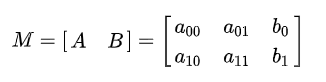根据旋转矩阵 A和平移矩阵B 以及图像像素值，仿射变换的数学原理可以用式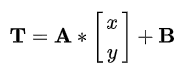仿射变换又称为三点变换，如果知道变换前后两张图像中三个像素点坐标的对应关系，就可以求得仿射变换中的变换矩阵 .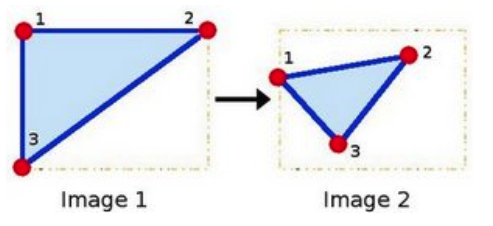点1, 2 和 3 (在图一中形成一个三角形) 与图二中三个点一一映射, 仍然形成三角形, 但形状已经大大改变. 如果我们能通过这样两组三点求出仿射变换 (你能选择自己喜欢的点), 接下来我们就能把仿射变换应用到图像中所有的点.。
而我们通常使用2 x 3的矩阵来表示仿射变换。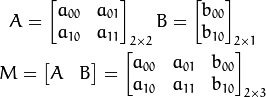opencv中仿射变换实现
要求出图像的仿射变换（旋转缩放等），首先要求出仿射变换矩阵。
使用getAffineTransform()函数求仿射变换矩阵
上文提到过，已知变换前后两张图像中三个像素点坐标的对应关系，就可以求得仿射变换中的变换矩阵。OpenCV 4提供了利用三个对应像素点来确定 矩阵的函数getAffineTransform()
函数原型：
CV_EXPORTS Mat getAffineTransform( const Point2f src[], const Point2f dst[] );

Calculates an affine transform from three pairs of the corresponding points. The function calculates the  matrix of an affine transform so that:

where

@param src Coordinates of triangle vertices in the source image. @param dst Coordinates of the corresponding triangle vertices in the destination image.
src[]：原图像中的三个像素坐标。dst[]：目标图像中的三个像素坐标。

使用 getRotationMatrix2D()函数获取仿射矩阵
函数原型：
CV_EXPORTS_W Mat getRotationMatrix2D(Point2f center, double angle, double scale);

/** @sa getRotationMatrix2D */
CV_EXPORTS Matx23d getRotationMatrix2D_(Point2f center, double angle, double scale);

inline
Mat getRotationMatrix2D(Point2f center, double angle, double scale)
{
return Mat(getRotationMatrix2D_(center, angle, scale), true);
}

@brief Calculates an affine matrix of 2D rotation.
The function calculates the following matrix:以此得出仿射矩阵：

where

The transformation maps the rotation center to itself. If this is not the target, adjust the shift.
@param center Center of the rotation in the source image. @param angle Rotation angle in degrees. Positive values mean counter-clockwise rotation (the coordinate origin is assumed to be the top-left corner). @param scale Isotropic scale factor.
第一个参数，Point2f类型的center，表示源图像的旋转中心。第二个参数，double类型的angle，旋转角度。角度为正值表示向逆时针旋转（坐标原点是左上角）。第三个参数，double类型的scale，缩放系数。

getRotationMatrix2D函数源代码：
cv::Mat cv::getRotationMatrix2D( Point2f center,double angle, double scale )
{
angle *= CV_PI/180;
double alpha = cos(angle)*scale;
double beta = sin(angle)*scale;

Mat M(2, 3, CV_64F);//生成一个2*3的矩阵
double* m = (double*)M.data;

//按照上文公式填充内容
m = alpha;
m = beta;
m = (1-alpha)*center.x - beta*center.y;
m = -beta;
m = alpha;
m = beta*center.x + (1-alpha)*center.y;

return M;
}

使用仿射矩阵对图像做仿射变换warpAffine
warpAffine函数原型：
CV_EXPORTS_W void warpAffine( InputArray src, OutputArray dst,
InputArray M, Size dsize,
int flags = INTER_LINEAR,
int borderMode = BORDER_CONSTANT,
const Scalar& borderValue = Scalar());

@brief Applies an affine transformation to an image.
The function warpAffine transforms the source image using the specified matrix:依据如下式子，对图像做仿射变换

when the flag #WARP_INVERSE_MAP is set. Otherwise, the transformation is first inverted with #invertAffineTransform and then put in the formula above instead of M. The function cannot operate in-place.
@param src input image. @param dst output image that has the size dsize and the same type as src . @param M  transformation matrix. @param dsize size of the output image. @param flags combination of interpolation methods (see #InterpolationFlags) and the optional flag #WARP_INVERSE_MAP that means that M is the inverse transformation (). @param borderMode pixel extrapolation method (see #BorderTypes); when borderMode=#BORDER_TRANSPARENT, it means that the pixels in the destination image corresponding to the "outliers" in the source image are not modified by the function. @param borderValue value used in case of a constant border; by default, it is 0.
第一个参数，InputArray类型的src，输入图像，即源图像，填Mat类的对象即可。第二个参数，OutputArray类型的dst，函数调用后的运算结果存在这里，需和源图片有一样的尺寸和类型。第三个参数，InputArray类型的M，2×3的变换矩阵。第四个参数，Size类型的dsize，表示输出图像的尺寸。第五个参数，int类型的flags，插值方法的标识符。此参数有默认值INTER_LINEAR(线性插值)，可选的插值方式如下：

INTER_NEAREST - 最近邻插值INTER_LINEAR - 线性插值（默认值）INTER_AREA - 区域插值INTER_CUBIC –三次样条插值INTER_LANCZOS4 -Lanczos插值CV_WARP_FILL_OUTLIERS - 填充所有输出图像的象素。如果部分象素落在输入图像的边界外，那么它们的值设定为 fillval.CV_WARP_INVERSE_MAP –表示M为输出图像到输入图像的反变换，即 。因此可以直接用来做象素插值。否则, warpAffine函数从M矩阵得到反变换。
第六个参数，int类型的borderMode，边界像素模式，默认值为BORDER_CONSTANT。第七个参数，const Scalar&类型的borderValue，在恒定的边界情况下取的值，默认值为Scalar()，即0。

使用两种方式进行仿射变换：
#include <opencv2\opencv.hpp>
#include <iostream>
#include <vector>

using namespace std;
using namespace cv;

int main()
{
if (img.empty())
{
cout << "请确认图像文件名称是否正确" << endl;
return -1;
}

Mat rotation0, rotation1, img_warp0, img_warp1;
double angle = 30;  //设置图像旋转的角度
Size dst_size(img.rows, img.cols);  //设置输出图像的尺寸
Point2f center(img.rows / 2.0, img.cols / 2.0);  //设置图像的旋转中心
rotation0 = getRotationMatrix2D(center, angle, 1);  //计算放射变换矩阵
warpAffine(img, img_warp0, rotation0, dst_size);  //进行仿射变换
imshow("img_warp0", img_warp0);
//根据定义的三个点进行仿射变换
Point2f src_points;
Point2f dst_points;
src_points = Point2f(0, 0);  //原始图像中的三个点
src_points = Point2f(0, (float)(img.cols - 1));
src_points = Point2f((float)(img.rows - 1), (float)(img.cols - 1));
//放射变换后图像中的三个点
dst_points = Point2f((float)(img.rows)*0.11, (float)(img.cols)*0.20);
dst_points = Point2f((float)(img.rows)*0.15, (float)(img.cols)*0.70);
dst_points = Point2f((float)(img.rows)*0.81, (float)(img.cols)*0.85);
rotation1 = getAffineTransform(src_points, dst_points);  //根据对应点求取仿射变换矩阵
warpAffine(img, img_warp1, rotation1, dst_size);  //进行仿射变换
imshow("img_warp1", img_warp1);
waitKey(0);
return 0;
}

实验效果：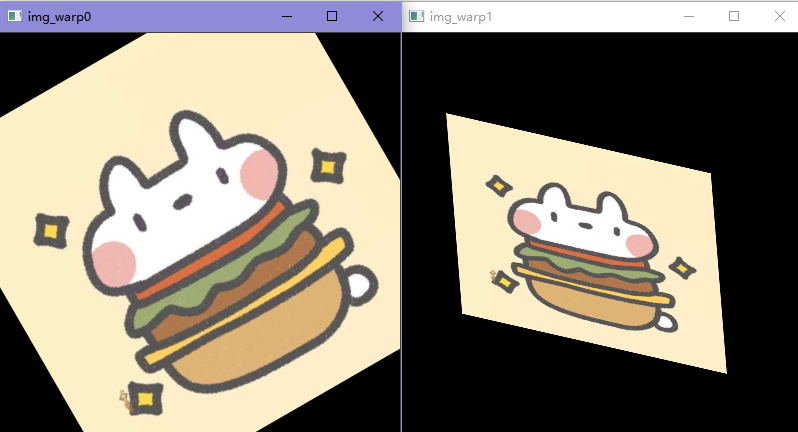参考文章：
【从零学习OpenCV 4】图像仿射变换
【OpenCV入门教程之十八】OpenCV仿射变换 & SURF特征点描述合辑
展开全文计算机视觉 opencv
• opencv 图像仿射变换 计算仿射变换后对应特征点的新坐标 图像旋转、缩放、平移 根据三点进行仿射变换
常常需要最图像进行仿射变换，仿射变换后，我们可能需要将原来图像中的特征点坐标进行重新计算，获得原来图像中例如眼睛瞳孔坐标的新的位置，用于在新得到图像中继续利用瞳孔位置坐标。

仿射变换在：http://blog.csdn.net/xiaowei_cqu/article/details/7616044 这位大牛的博客中已经介绍的非常清楚。
关于仿射变换的详细介绍，请见上面链接的博客。
我这里主要介绍如何在已经知道原图像中若干特征点的坐标之后，计算这些特征点进行放射变换之后的坐标，然后做一些补充。

** 在原文中，很多功能函数都是使用的cvXXX，例如cv2DRotationMatrix( center, degree,1, &M);  这些都是老版本的函数，在opencv2以后，应该尽量的使用全新的函数，所以在我的代码中，都是使用的最新的函数，不再使用 cvMat， 而是全部使用 Mat 类型。 **

1. 特征点对应的新的坐标计算
假设已经有一个原图像中的特征点的坐标 CvPoint point;  那么计算这个point的对应的仿射变换之后在新的图像中的坐标位置，使用的方法如下函数：

// 获取指定像素点放射变换后的新的坐标位置
CvPoint getPointAffinedPos(const CvPoint &src, const CvPoint ¢er, double angle)
{
CvPoint dst;
int x = src.x - center.x;
int y = src.y - center.y;

dst.x = cvRound(x * cos(angle) + y * sin(angle) + center.x);
dst.y = cvRound(-x * sin(angle) + y * cos(angle) + center.y);
return dst;
}
要特别注意的是，在对一个原图像中的像素的坐标进行计算仿射变换之后的坐标的时候，一定要按照仿射变换的基本原理，将原来的坐标减去仿射变换的旋转中心的坐标，这样仿射变换之后得到的坐标再加上仿射变换旋转中心坐标才是原坐标在新的仿射变换之后的图像中的正确坐标。

下面给出计算对应瞳孔坐标旋转之后的坐标位置的示例代码：

// AffineTransformation.cpp : Defines the entry point for the console application.
//

#include "stdafx.h"
#include "stdio.h"
#include "iostream"

#include "opencv2/opencv.hpp"

using namespace std;
using namespace cv;

// 获取指定像素点放射变换后的新的坐标位置
CvPoint getPointAffinedPos(const CvPoint &src, const CvPoint ¢er, double angle);
Mat ImageRotate(Mat & src, const CvPoint &_center, double angle);
Mat ImageRotate2NewSize(Mat& src, const CvPoint &_center, double angle);

int _tmain(int argc, _TCHAR* argv[])
{
string image_path = "D:/lena.jpg";
cvtColor(img, img, CV_BGR2GRAY);

Mat src;
img.copyTo(src);

CvPoint Leye;
Leye.x = 265;
Leye.y = 265;
CvPoint Reye;
Reye.x = 328;
Reye.y = 265;

// draw pupil
src.at<unsigned char>(Leye.y, Leye.x) = 255;
src.at<unsigned char>(Reye.y, Reye.x) = 255;

imshow("src", src);

//
CvPoint center;
center.x = img.cols / 2;
center.y = img.rows / 2;

double angle = 15L;

Mat dst = ImageRotate(img, center, angle);

// 计算原特征点在旋转后图像中的对应的坐标
CvPoint l2 = getPointAffinedPos(Leye, center, angle * CV_PI / 180);
CvPoint r2 = getPointAffinedPos(Reye, center, angle * CV_PI / 180);

// draw pupil
dst.at<unsigned char>(l2.y, l2.x) = 255;
dst.at<unsigned char>(r2.y, r2.x) = 255;

//Mat dst = ImageRotate2NewSize(img, center, angle);
imshow("dst", dst);

waitKey(0);
return 0;
}

Mat ImageRotate(Mat & src, const CvPoint &_center, double angle)
{
CvPoint2D32f center;
center.x = float(_center.x);
center.y = float(_center.y);

//计算二维旋转的仿射变换矩阵
Mat M = getRotationMatrix2D(center, angle, 1);

// rotate
Mat dst;
warpAffine(src, dst, M, cvSize(src.cols, src.rows), CV_INTER_LINEAR);
return dst;
}

// 获取指定像素点放射变换后的新的坐标位置
CvPoint getPointAffinedPos(const CvPoint &src, const CvPoint ¢er, double angle)
{
CvPoint dst;
int x = src.x - center.x;
int y = src.y - center.y;

dst.x = cvRound(x * cos(angle) + y * sin(angle) + center.x);
dst.y = cvRound(-x * sin(angle) + y * cos(angle) + center.y);
return dst;
}
这里，我们先通过手工找到瞳孔坐标，然后计算在图像旋转之后瞳孔的坐标。

运行结果如图：
原图像旋转之后的图像：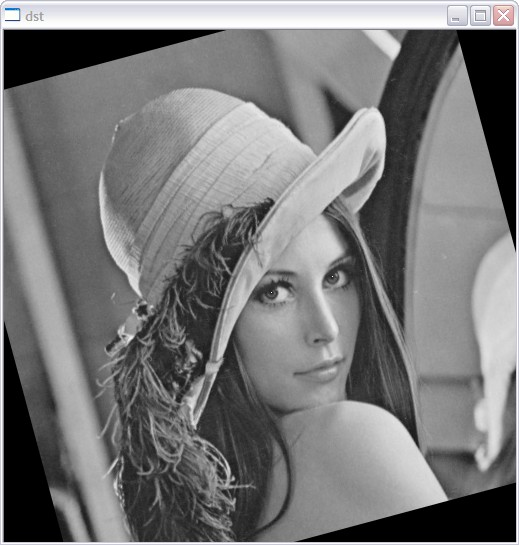2. 旋转中心对于旋转的影响
然后我们看看仿射变换旋转点的选择对于旋转之后的图像的影响，一般情况下，我们选择图像的中心点作为仿射变换的旋转中心，获得的旋转之后的图像与原图像大小一样。
计算代码：

int _tmain(int argc, _TCHAR* argv[])
{
string image_path = "D:/lena.jpg";
cvtColor(img, img, CV_BGR2GRAY);

Mat src;
img.copyTo(src);

CvPoint Leye;
Leye.x = 265;
Leye.y = 265;
CvPoint Reye;
Reye.x = 328;
Reye.y = 265;

// draw pupil
src.at<unsigned char>(Leye.y, Leye.x) = 255;
src.at<unsigned char>(Reye.y, Reye.x) = 255;

imshow("src", src);

//
/*CvPoint center;
center.x = img.cols / 2;
center.y = img.rows / 2;*/
CvPoint center;
center.x = 0;
center.y = 0;

double angle = 15L;

Mat dst = ImageRotate(img, center, angle);

// 计算原特征点在旋转后图像中的对应的坐标
CvPoint l2 = getPointAffinedPos(Leye, center, angle * CV_PI / 180);
CvPoint r2 = getPointAffinedPos(Reye, center, angle * CV_PI / 180);

// draw pupil
dst.at<unsigned char>(l2.y, l2.x) = 255;
dst.at<unsigned char>(r2.y, r2.x) = 255;

//Mat dst = ImageRotate2NewSize(img, center, angle);
imshow("dst", dst);

waitKey(0);
return 0;
}
这里绕着（0,0）点进行旋转，旋转之后的图像：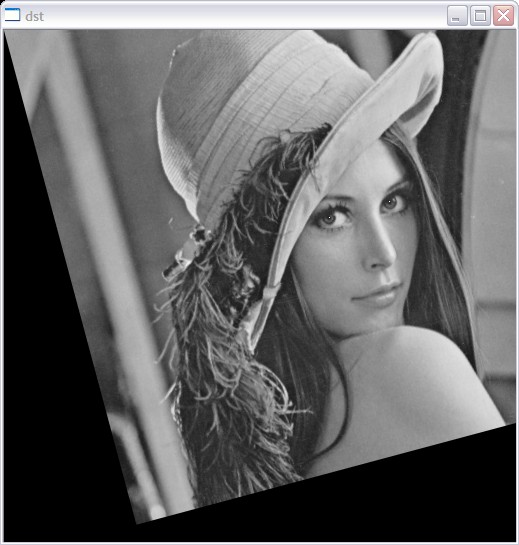绕着左下角旋转：

	CvPoint center;
center.x = 0;
center.y = img.rows;

旋转之后的图像：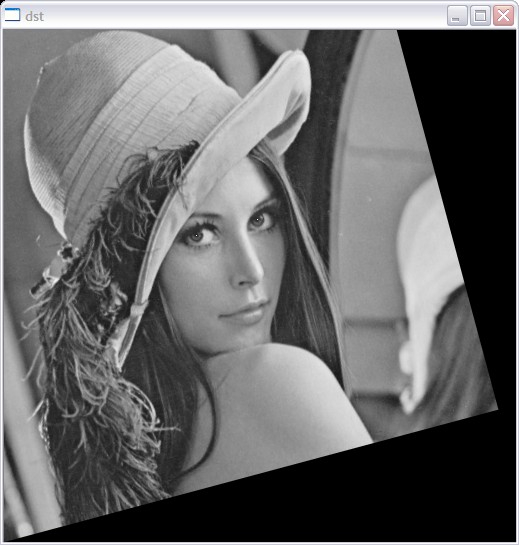3. 缩放因子对于旋转图像的影响
上面我们的代码都没有添加缩放信息，现在对上面的代码进行稍加修改，添加缩放参数，然后看一下如何计算对应的新的坐标。

#include "stdafx.h"
#include "stdio.h"
#include "iostream"

#include "opencv2/opencv.hpp"

using namespace std;
using namespace cv;

// 获取指定像素点放射变换后的新的坐标位置
CvPoint getPointAffinedPos(const CvPoint &src, const CvPoint ¢er, double angle, double scale);
Mat ImageRotate(Mat & src, const CvPoint &_center, double angle, double scale);
Mat ImageRotate2NewSize(Mat& src, const CvPoint &_center, double angle, double scale);

int _tmain(int argc, _TCHAR* argv[])
{
string image_path = "D:/lena.jpg";
cvtColor(img, img, CV_BGR2GRAY);
double scale = 0.5;

Mat src;
img.copyTo(src);

CvPoint Leye;
Leye.x = 265;
Leye.y = 265;
CvPoint Reye;
Reye.x = 328;
Reye.y = 265;

// draw pupil
src.at<unsigned char>(Leye.y, Leye.x) = 255;
src.at<unsigned char>(Reye.y, Reye.x) = 255;

imshow("src", src);

//
CvPoint center;
center.x = img.cols / 2;
center.y = img.rows / 2;

double angle = 15L;

Mat dst = ImageRotate(img, center, angle, scale);

// 计算原特征点在旋转后图像中的对应的坐标
CvPoint l2 = getPointAffinedPos(Leye, center, angle * CV_PI / 180, scale);
CvPoint r2 = getPointAffinedPos(Reye, center, angle * CV_PI / 180, scale);

// draw pupil
dst.at<unsigned char>(l2.y, l2.x) = 255;
dst.at<unsigned char>(r2.y, r2.x) = 255;

//Mat dst = ImageRotate2NewSize(img, center, angle);
imshow("dst", dst);

waitKey(0);
return 0;
}

Mat ImageRotate(Mat & src, const CvPoint &_center, double angle, double scale)
{
CvPoint2D32f center;
center.x = float(_center.x);
center.y = float(_center.y);

//计算二维旋转的仿射变换矩阵
Mat M = getRotationMatrix2D(center, angle, scale);

// rotate
Mat dst;
warpAffine(src, dst, M, cvSize(src.cols, src.rows), CV_INTER_LINEAR);
return dst;
}

// 获取指定像素点放射变换后的新的坐标位置
CvPoint getPointAffinedPos(const CvPoint &src, const CvPoint ¢er, double angle, double scale)
{
CvPoint dst;
int x = src.x - center.x;
int y = src.y - center.y;

dst.x = cvRound(x * cos(angle) * scale  + y * sin(angle) * scale + center.x);
dst.y = cvRound(-x * sin(angle) * scale + y * cos(angle) * scale + center.y);
return dst;
}
当缩放尺度为0.5的时候，程序的运行结果如图：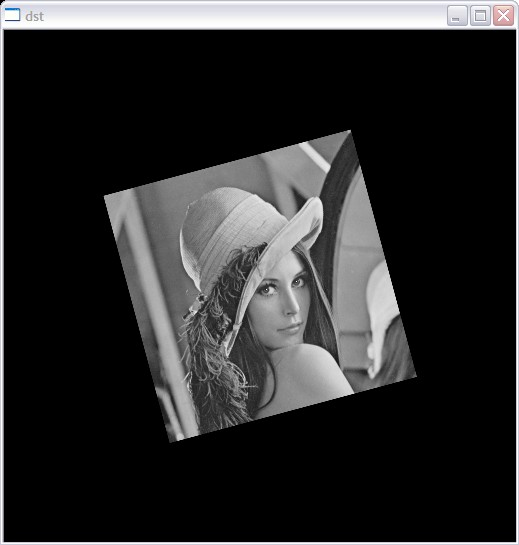4.  根据旋转与缩放尺度获得与原始图像大小不同的图像大小（新的合适的大小）

上面的计算中，一直都是放射变换之后计算得到的图像和原始图像一样大，但是因为旋转、缩放之后图像可能会变大或者变小，我们再次对上面的代码进行修改，这样在获得仿射变换之后的图像前，需要重新计算生成的图像的大小。

计算方法：

	double angle2 = angle * CV_PI / 180;
int width = src.cols;
int height = src.rows;

double alpha = cos(angle2) * scale;
double beta = sin(angle2) * scale;

int new_width = (int)(width * fabs(alpha) + height * fabs(beta));
int new_height = (int)(width * fabs(beta) + height * fabs(alpha));

另外，因为我们的图像旋转是按照原图像的中心，所以当获取到图像的仿射变换矩阵之后，我们需要根据新生成的图像的大小，给仿射变换矩阵添加平移信息。

或者可以这么说，我们新计算得到的图像的大小，让原始图像绕着新的图像大小的中心进行旋转。

	//计算二维旋转的仿射变换矩阵
Mat M = getRotationMatrix2D(center, angle, scale);

// 给计算得到的旋转矩阵添加平移

M.at<double>(0, 2) += (int)((new_width - width )/2);
M.at<double>(1, 2) += (int)((new_height - height )/2);

然后另外需要注意的是，如果你在原始图像中有一些特征点的坐标，这些特征点的坐标映射到新的图像上的时候，需要在以前的方法的基础上增加平移信息。

// 获取指定像素点放射变换后的新的坐标位置
CvPoint getPointAffinedPos(Mat & src, Mat & dst, const CvPoint &src_p, const CvPoint ¢er, double angle, double scale)
{
double alpha = cos(angle) * scale;
double beta = sin(angle) * scale;

int width = src.cols;
int height = src.rows;

CvPoint dst_p;
int x = src_p.x - center.x;
int y = src_p.y - center.y;

dst_p.x = cvRound(x * alpha  + y * beta + center.x);
dst_p.y = cvRound(-x * beta + y * alpha + center.y);

int new_width = dst.cols;
int new_height = dst.rows;

int movx = (int)((new_width - width)/2);
int movy = (int)((new_height - height)/2);

dst_p.x += movx;
dst_p.y += movy;

return dst_p;
}

我们仿射变换函数代码：

Mat ImageRotate2NewSize(Mat& src, const CvPoint &_center, double angle, double scale)
{
double angle2 = angle * CV_PI / 180;
int width = src.cols;
int height = src.rows;

double alpha = cos(angle2) * scale;
double beta = sin(angle2) * scale;

int new_width = (int)(width * fabs(alpha) + height * fabs(beta));
int new_height = (int)(width * fabs(beta) + height * fabs(alpha));

CvPoint2D32f center;
center.x = float(width / 2);
center.y = float(height / 2);
//计算二维旋转的仿射变换矩阵
Mat M = getRotationMatrix2D(center, angle, scale);

// 给计算得到的旋转矩阵添加平移

M.at<double>(0, 2) += (int)((new_width - width )/2);
M.at<double>(1, 2) += (int)((new_height - height )/2);

// rotate
Mat dst;
warpAffine(src, dst, M, cvSize(new_width, new_height), CV_INTER_LINEAR);
return dst;
}
主函数：

int _tmain(int argc, _TCHAR* argv[])
{
string image_path = "D:/lena.jpg";
cvtColor(img, img, CV_BGR2GRAY);
double scale = 0.5;

Mat src;
img.copyTo(src);

CvPoint Leye;
Leye.x = 265;
Leye.y = 265;
CvPoint Reye;
Reye.x = 328;
Reye.y = 265;

// draw pupil
src.at<unsigned char>(Leye.y, Leye.x) = 255;
src.at<unsigned char>(Reye.y, Reye.x) = 255;

imshow("src", src);

//
CvPoint center;
center.x = img.cols / 2;
center.y = img.rows / 2;

double angle = 15L;

//Mat dst = ImageRotate(img, center, angle, scale);
Mat dst = ImageRotate2NewSize(img, center, angle, scale);

// 计算原特征点在旋转后图像中的对应的坐标
CvPoint l2 = getPointAffinedPos(src, dst, Leye, center, angle * CV_PI / 180, scale);
CvPoint r2 = getPointAffinedPos(src, dst, Reye, center, angle * CV_PI / 180, scale);

// draw pupil
dst.at<unsigned char>(l2.y, l2.x) = 255;
dst.at<unsigned char>(r2.y, r2.x) = 255;

imshow("dst", dst);

waitKey(0);
return 0;
}
仿射变换结果以及瞳孔重新坐标计算结果：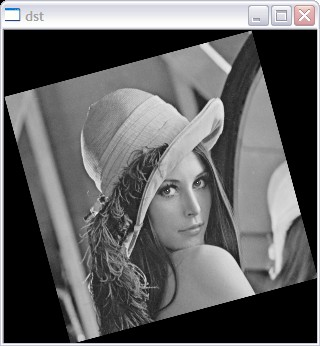5. 根据三个点进行仿射变换

根据给点的三个点，由这三个点之前的坐标以及变换之后的坐标，对原图像进行仿射变换，不过需要事先知道三个点仿射变换的坐标位置。

int _tmain(int argc, _TCHAR* argv[])
{
string image_path = "D:/lena.jpg";

Point2f src_points;
src_points = Point2f(100, 100);
src_points = Point2f(400, 100);
src_points = Point2f(250, 300);

Point2f dst_points;
dst_points = Point2f(100, 100);
dst_points = Point2f(400, 300);
dst_points = Point2f(100, 300);

Mat M1 = getAffineTransform(src_points, dst_points);

Mat dst;
warpAffine(img, dst, M1, cvSize(img.cols, img.rows), INTER_LINEAR);

imshow("dst", dst);

//cvtColor(img, img, CV_BGR2GRAY);
//double scale = 1.5;

//Mat src;
//img.copyTo(src);

//CvPoint Leye;
//Leye.x = 265;
//Leye.y = 265;
//CvPoint Reye;
//Reye.x = 328;
//Reye.y = 265;

draw pupil
//src.at<unsigned char>(Leye.y, Leye.x) = 255;
//src.at<unsigned char>(Reye.y, Reye.x) = 255;

//imshow("src", src);

//CvPoint center;
//center.x = img.cols / 2;
//center.y = img.rows / 2;

//double angle = 15L;

Mat dst = ImageRotate(img, center, angle, scale);
//Mat dst = ImageRotate2NewSize(img, center, angle, scale);

计算原特征点在旋转后图像中的对应的坐标
//CvPoint l2 = getPointAffinedPos(src, dst, Leye, center, angle * CV_PI / 180, scale);
//CvPoint r2 = getPointAffinedPos(src, dst, Reye, center, angle * CV_PI / 180, scale);

draw pupil
//dst.at<unsigned char>(l2.y, l2.x) = 255;
//dst.at<unsigned char>(r2.y, r2.x) = 255;
//imshow("dst", dst);

waitKey(0);
return 0;
}
结果：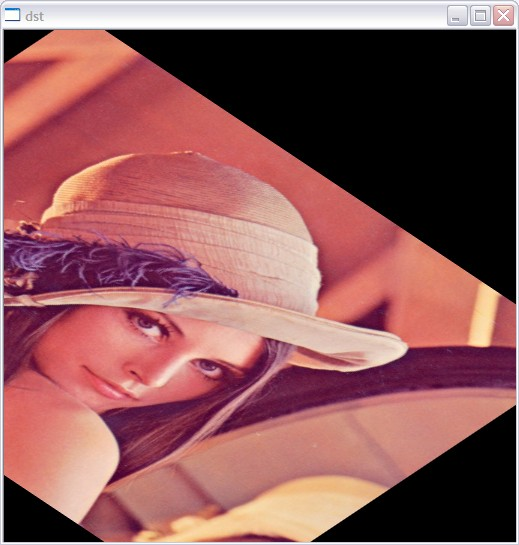展开全文• 利用相位相关算法计算两张图的平移距离 2.相位相关方法应用简介 相位相关方法基于频率域, 利用傅里叶变换将图像信息从空间域变换到频率域后, 获取图像的变换关系。该方法根据互功率谱相位信息求取图像间的相对偏移量...相位相关
• 在我们读计算机视觉的相关论文时，经常会看到平移不变性这词，通常观点中，大家都是普遍认为CNN是具有平移不变性的，然而近年来，有一些工作的研究对平移不变性提出了质疑，本文对一些平移不变性的理解进行记录，...
• CNN是否存在平移和尺度的不变性和相等性 ref https://zhuanlan.zhihu.com/p/113443895 论文：How much position information do convolutional neural networks encode? 图中一共有组图片，每组由原图和剪切图构成...
• 典型的变换包括缩小放大与平移。 （一）缩放 （见前一篇文章） （二）平移 为了实现平移，这里以按下鼠标中间键并移动鼠标作为事件触发方式，来实现平移。即先下辖鼠标中键（滚轮键），移动鼠标，这样WPF元素就...WPF Canvas
• 直方图平移的可逆信息隐藏 摘要：可逆数据隐藏（RDH）也被称为无损或可逆数据隐藏，已逐渐成为数据隐藏领域非常活跃的研究领域。本文介绍了多种基于直方图平移的可逆信息隐藏算法，并对各种算法的技术发展历史，现状...算法 矩阵
• SVG概述：SVG是可缩放矢量图形(Scalable Vector Graphics)，它基于可...百度上有SVG的一大堆介绍，这里就不细细说明，仅摘出重点，SVG主要特点是： 不失真——因为是矢量图 支持任意缩放——可用于地图存储 存储介...svg html
• 但是可以看Rotation向量，计算出来的值似乎是显示所有的旋转都是绕着第三个量也就是Z轴进行的，而在实际过程中，旋转似乎是主要绕着Y轴进行的。 当然根据这个结果我们可以大致绘制出机器人的行进路线图，背后的...reference 嵌入式 null
• 注意，这里的平移旋转是将当前绘图坐标系看做一整体在世界坐标系中进行旋转平移。然后，改变以后，再用glVertex3f()等绘图函数绘图时，都是在当前绘图坐标系进行绘图，所有的函数参数也都是相对当前绘图坐标系来讲...opengl
• 几何空间变换是图像处理中的最基础的算法，主要包括图像的旋转，平移，缩放，偏移，组合变换等等，在冈萨雷斯的数字图像处理第版的第二章就做了相关介绍，最常用的空间坐标变换之一就是仿射变换。虽然仿射变换很...图像处理算法 仿射变换 c++ opencv
• 计算机图形学，输入的是结构特征数据，输出的是图像信号； 计算机视觉，输入的是图像信号，输出的是结构特征数据； 数字图像处理，输入的是图像信号，输出的也是图像信号。 计算机图形系统的组成 从计算机图形系统的...
• Unity3D 游戏引擎之脚本实现模型的平移与旋转雨松MOMO原创文章如转载，请注明：转载至我的独立域名博客雨松MOMO程序研究院，原文地址:http://www.xuanyusong.com/archives/501这一章MOMO带大家讨论一下Unity3D中使用...脚本 引擎 function
• 根据上面的特点，可以计算变化后的模型的维坐标。 对于下图的包含关系（ MatrixTransform A中包含一 MatrixTransform B， MatrixTransform B中包含一模型 ） 那么,模型的新坐标 (X, Y, Z) = ...matrix
• 作为一强大的科学计算软件，MATLAB广泛运用于较多领域，以其简单的编程风格著称。这篇文章便通过matlab语言来讲述如何进行图像的各种几何变换。 图像几何变换又称为图像空间变换，它是将一幅图像中的坐标位置，...
• 但是可以看Rotation向量，计算出来的值似乎是显示所有的旋转都是绕着第三个量也就是Z轴进行的，而在实际过程中，旋转似乎是主要绕着Y轴进行的。 当然根据这个结果我们可以大致绘制出机器人的行进路线图，背后的...reference matrix 嵌入式 null
• * 迭代最近点 Iterative Closest Point, ICP求解 3D坐标 到 3D坐标的转换矩阵(不用求解距离 激光SLAM 以及 RGB-D SLAM) ...* 使用 线性代数SVD奇异值分解 或者 非线性优化方法 求解 ...* 特征点匹配 得SVD
• * 或则 双目相机 深度相机可以直接得到 维点 不需要初始化得到最开始的维点 *可使用3D点-2D点对 使用 直接线性变换DLT(Direct Linear Transform) n点透视问题(PnP) 最小二乘最小化重投影误差 ...
• 而运用三个kinect来进行人体建模，只需要将三个kinect按照一定角度（比如各相距120°）固定位置，人体不动也能实现对人体的全身扫描，相比之下时间花销短。  基于三个kinect的人体建模，首先要对三个kineckinect
• 正射投影（简称投影）是常见的一种线性变换，不过通常它会改变向量的长度，因此它不是一种正交... 在计算投影向量时，如果要在任意平面上投影，可以事先将平面平移到原点，计算完毕后再平移回去。 设维空间中过原...
• 平稳性的实质是对时间平移的不变性做了假设，有了这种假设通过历史数据去预测未来才有了基础，否则历史数据的统计特征对未来预测毫无作用。 强平稳是事实上的平稳，它要求时间序列的点同分布，它过于严格而且......